## Thursday, April 20, 2017

### Extracting the positions of character state changes from stochastic map trees in phytools

Yesterday, a friend & colleague emailed me the following question:

“Me gustaría preguntarte si se pueden extraer las fechas estimadas de las transiciones de estado en un análisis SIMMAP en phytools?”

that is:

“I'd like to ask you if one can extract the estimated dates of state transition in a stochastic mapping (SIMMAP) analysis in phytools?”

The answer is “yes” - using the function `markChanges`. For instance:

``````library(phytools)
tree
``````
``````##
## Phylogenetic tree with 26 tips and 25 internal nodes.
##
## Tip labels:
##  A, B, C, D, E, F, ...
##
## Rooted; includes branch lengths.
``````
``````x
``````
``````## A B C D E F G H I J K L M N O P Q R S T U V W X Y Z
## b b a a b a b b a a a a a a a a b b b b b b b a a b
## Levels: a b
``````
``````mtree<-make.simmap(tree,x)
``````
``````## make.simmap is sampling character histories conditioned on the transition matrix
##
## Q =
##            a          b
## a -0.9111912  0.9111912
## b  0.9111912 -0.9111912
## (estimated using likelihood);
## and (mean) root node prior probabilities
## pi =
##   a   b
## 0.5 0.5
``````
``````## Done.
``````
``````cols<-setNames(c("blue","red"),c("a","b"))
plot(mtree,cols,ftype="i",lwd=3,mar=c(4.1,1.1,1.1,1.1))
axis(1)
obj<-markChanges(mtree,colors=cols,lwd=3)
``````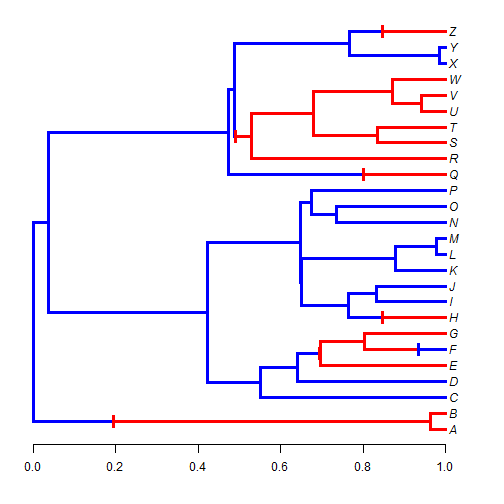Here, I've marked the sampled changes on the tree - but I've also extracted their positions above the root in the matrix `obj`:

``````obj
``````
``````##              x       y
## a->b 0.1955114  1.5000
## a->b 0.6950576  5.7500
## b->a 0.9342177  6.0000
## a->b 0.8472460  8.0000
## a->b 0.8019033 17.0000
## a->b 0.4896849 19.4375
## a->b 0.8475341 26.0000
``````

We can see this if we drop our marked changes to the x-axis:

``````plot(mtree,cols,ftype="i",lwd=3,mar=c(4.1,1.1,1.1,1.1))
axis(1)
obj<-markChanges(mtree,plot=FALSE)
for(i in 1:nrow(obj)) lines(rep(obj[i,1],2),c(0,obj[i,2]),lty="dotted",
col=make.transparent("grey",0.8))
points(obj[,1],rep(0.2,nrow(obj)),pch=21,bg="grey")
markChanges(mtree,cols,lwd=3)
``````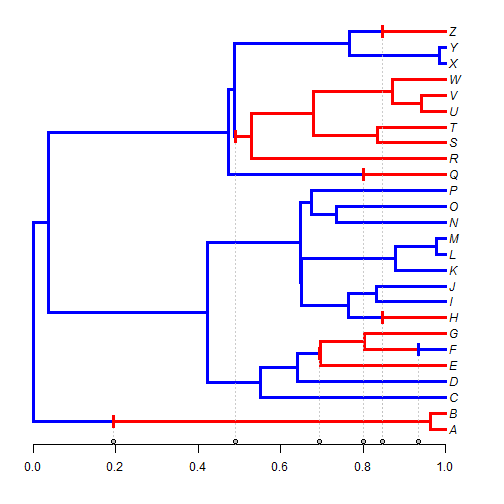Normally, however, we should probably obtain a set of trees from the posterior distribution - rather than a single tree. For instance:

``````mtrees<-make.simmap(tree,x,nsim=100)
``````
``````## make.simmap is sampling character histories conditioned on the transition matrix
##
## Q =
##            a          b
## a -0.9111912  0.9111912
## b  0.9111912 -0.9111912
## (estimated using likelihood);
## and (mean) root node prior probabilities
## pi =
##   a   b
## 0.5 0.5
``````
``````## Done.
``````

Just a reminder that phytools already includes a cool S3 `density` function for the number of changes of different types in an object of class `"multiSimmap"` consisting of a posterior sample of trees:

``````obj<-density(mtrees)
obj
``````
``````##
## Distribution of changes from stochastic mapping:
##  a->b        b->a
##  Min.   :1   Min.   :1
##  Median :5   Median :3
##  Mean   :4.81    Mean   :3.46
##  Max.   :9   Max.   :7
##
## 95% HPD interval(a->b): [1, 6]
## 95% HPD interval(b->a): [1, 6]
``````
``````plot(obj)
``````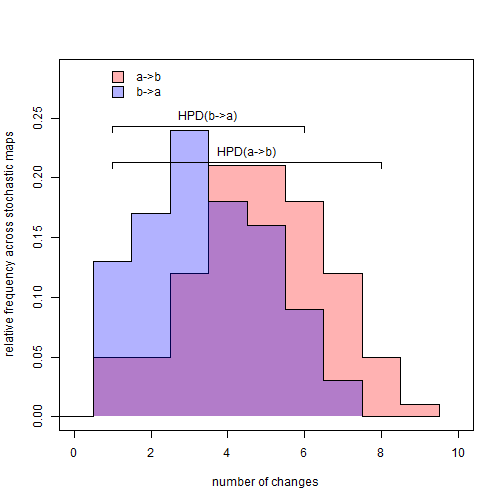We can also try to get a posterior density on the positions of these various changes between states:

``````dotTree(tree,x,colors=cols,ftype="i",lwd=3,mar=c(4.1,1.1,1.1,1.1))
axis(1)
obj<-sapply(mtrees,markChanges,colors=sapply(cols,make.transparent,alpha=0.1))
make.transparent,0.4),prompt=FALSE,x=0,y=26,vertical=TRUE)
``````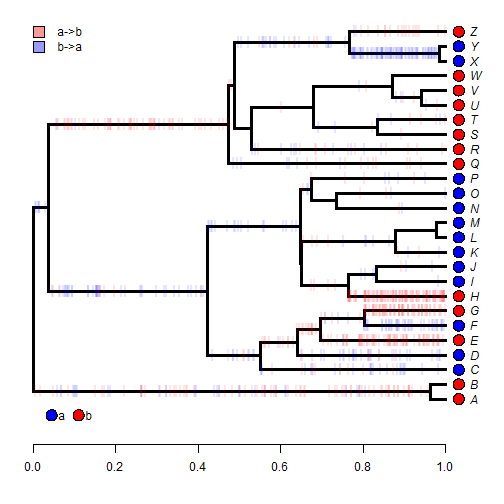This is just a list of matrices. For instance, here are the first 10:

``````obj[1:10]
``````
``````## []
##              x        y
## b->a 0.2636368  8.34375
## a->b 0.7965588  5.00000
## a->b 0.9748351  7.00000
## a->b 0.9750953  8.00000
## b->a 0.3070973 19.67188
## a->b 0.4710849 19.67188
## b->a 0.9217101 24.50000
##
## []
##               x        y
## b->a 0.08406267  8.34375
## a->b 0.70315021  5.00000
## a->b 0.89604958  7.00000
## a->b 0.76897714  8.00000
## b->a 0.67039182 25.25000
## a->b 0.68162989 25.25000
## b->a 0.96638624 24.50000
##
## []
##               x        y
## a->b 0.37282213  1.50000
## b->a 0.61444432  1.50000
## a->b 0.88630420  1.50000
## a->b 0.87318915  3.00000
## b->a 0.94439736  3.00000
## a->b 0.89377892  5.00000
## a->b 0.81464365  7.00000
## a->b 0.91710938  8.00000
## a->b 0.07535913 19.67188
## b->a 0.89555680 24.50000
##
## []
##              x        y
## b->a 0.2229673  8.34375
## a->b 0.7156098  5.00000
## a->b 0.9524018  7.00000
## a->b 0.8485678  8.00000
## b->a 0.8271941 24.50000
##
## []
##              x        y
## a->b 0.7918600  1.50000
## a->b 0.8387755  5.00000
## a->b 0.9637919  7.00000
## a->b 0.8172940  8.00000
## a->b 0.6557096 15.25000
## b->a 0.6655496 15.25000
## a->b 0.2856692 19.67188
## b->a 0.8764252 24.50000
##
## []
##              x        y
## a->b 0.5567777  1.50000
## a->b 0.9484923  5.00000
## a->b 0.9339515  7.00000
## a->b 0.8220921  8.00000
## a->b 0.2525068 19.67188
## b->a 0.7149046 25.25000
## a->b 0.9215291 26.00000
##
## []
##              x        y
## a->b 0.3328872  1.50000
## a->b 0.6776737  5.75000
## b->a 0.7405165  6.50000
## a->b 0.7941714  6.50000
## b->a 0.8458889  6.00000
## a->b 0.9726573  8.00000
## a->b 0.2351046 19.67188
## b->a 0.9340201 24.50000
##
## []
##               x        y
## a->b 0.45241952  1.50000
## a->b 0.04991146  8.34375
## b->a 0.15720353  8.34375
## a->b 0.67075616  5.75000
## b->a 0.86765865  6.00000
## a->b 0.99840460  8.00000
## a->b 0.29504460 19.67188
## b->a 0.71119438 25.25000
## a->b 0.92642095 26.00000
##
## []
##              x        y
## b->a 0.3217994  8.34375
## a->b 0.8831721  5.00000
## a->b 0.8332154  7.00000
## a->b 0.9316393  8.00000
## b->a 0.8609643 24.50000
##
## []
##              x        y
## b->a 0.3951734  1.50000
## a->b 0.8865010  1.50000
## b->a 0.4090050  8.34375
## a->b 0.9239062  5.00000
## a->b 0.9447590  7.00000
## a->b 0.9709664  8.00000
## b->a 0.6098279 25.25000
## a->b 0.6222670 25.25000
## b->a 0.9539116 24.50000
``````

It seems like we should first accumulate the x column of all these matrices into a single vector:

``````X<-unlist(sapply(obj,function(x) x[,1]))
``````

Now the changes of each type:

``````transitions<-sort(unique(names(X)))
heights<-lapply(transitions,function(n,x) x[which(names(x)==n)],x=X)
obj<-lapply(heights,hist,plot=FALSE)
par(mfrow=c(2,1))
barplot(obj[]\$counts/length(mtrees),space=0,cex.names=0.75,
names.arg=paste(obj[]\$breaks[1:10],obj[]\$breaks[2:11],sep="-"),
main=paste("transitions",names(heights[])),
ylab=paste("average number of changes",names(heights[])),
xlab="height above the root")
axis(1,at=1:10-0.5,labels=rep("",10))
barplot(obj[]\$counts/length(mtrees),space=0,cex.names=0.75,
names.arg=paste(obj[]\$breaks[1:10],obj[]\$breaks[2:11],sep="-"),
main=paste("transitions",names(heights[])),
ylab=paste("average number of changes",names(heights[])),
xlab="height above the root")
axis(1,at=1:10-0.5,labels=rep("",10))
``````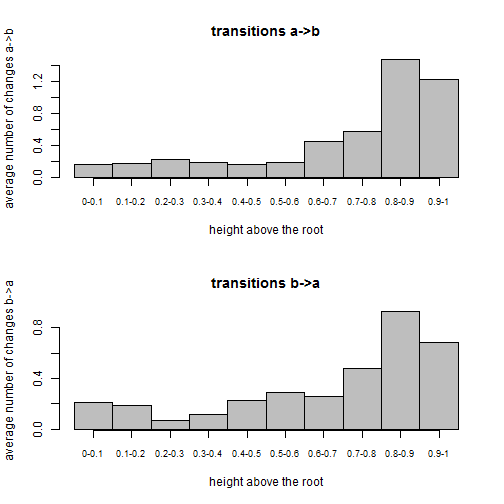Note that there is a lot more edge lengths towards the tips of the tree - which explains why we tend to see a lot more reconstructed changes in that region.

This example uses a binary character, but it could easily be extended (with some modifications, obviously) to a multistate character.

The data & tree were simulated as follows:

``````Q<-matrix(c(-1,1,1,-1),2,2)
rownames(Q)<-colnames(Q)<-letters[1:2]
x<-as.factor(sim.history(tree<-pbtree(n=26,tip.label=LETTERS,scale=1),Q)\$states)
``````

1.Hi Liam,

Thanks for the great resource! I've been trying to extract the distribution of state changes following the code you've presented on this page but I get the following error message:

> obj<-density(mtrees)
Error in density.default(mtrees) : argument 'x' must be numeric

Everything works great up until that point. Any idea what my problem is? Here's the preceding code and output, if that makes a difference:

> mtrees<-make.simmap(tree2,states2,nsim=100)
make.simmap is sampling character histories conditioned on the transition matrix

Q =
1 2 3
1 -0.014773612 0.01228084 0.002492774
2 0.012280838 -0.02237385 0.010093012
3 0.002492774 0.01009301 -0.012585787
(estimated using likelihood);
and (mean) root node prior probabilities
pi =
1 2 3
0.3333333 0.3333333 0.3333333
Done.

1.What version of phytools are you using? You may need to update the package.

2.3.